#### How to assess and repair probability miscalibration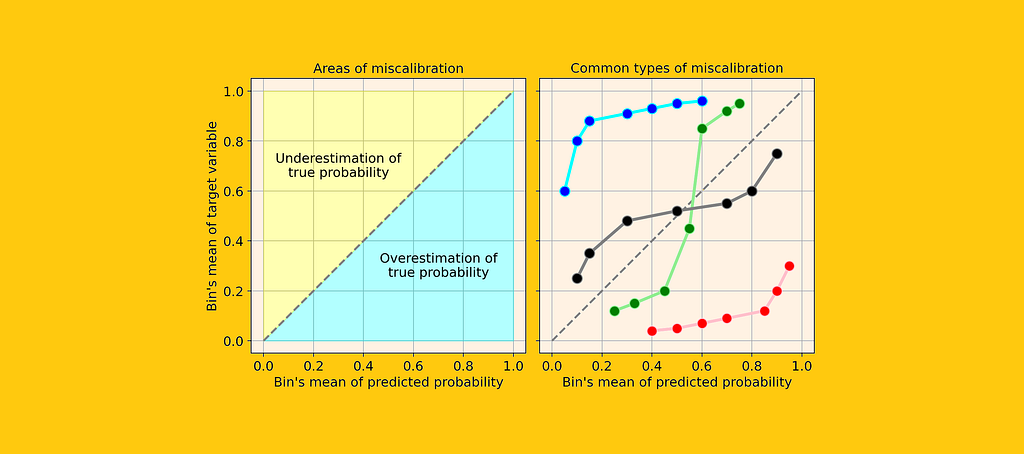[Image by Author]

Data scientists typically evaluate their predictive models in terms of accuracy or precision, but hardly ever ask themselves:

“Is my model capable of predicting real probabilities?”

However, an accurate estimate of probability is extremely valuable from a business point of view (sometimes even more valuable than a good precision). Want an example?

Imagine your company is selling 2 mugs, one is a plain white mug, and the other one has a kitten picture on it. You must decide which mug to show to a given customer. In order to do that, you need to predict the probability that a given user will buy each of them. So you train a couple of different models and you get these results: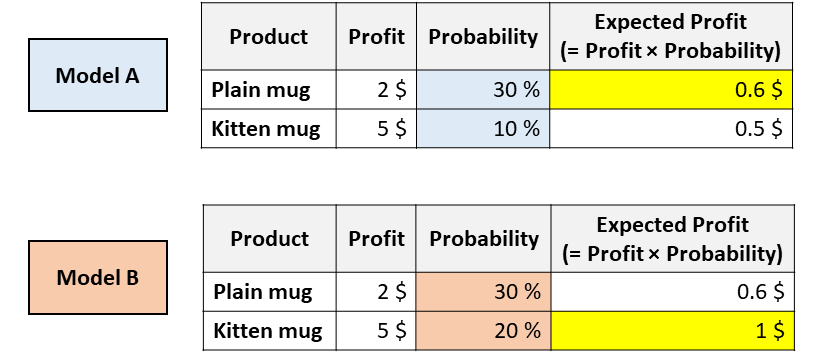Models with same ROC but different calibration. [Image by Author]

Now, which mug would you suggest to this user?

Both models agree that the user is more likely to buy the plain mug (so, model A and model B have the same area under ROC, since this metric only evaluates the sorting).

But, according to model A, you will maximize the expected profit by recommending the plain mug. Whereas, according to model B the expected profit is maximized by the kitten mug.

In applications like this one, it’s vital to figure out which model is able to estimate better probabilities.

In this article, we will see how to measure probability calibration (both visually and numerically) and how to “correct” an existing model in order to get better probabilities.

### What’s wrong with «predict_proba»

All the most popular machine learning libraries in Python have a method called «predict_proba»: Scikit-learn (e.g. LogisticRegression, SVC, RandomForest, …), XGBoost, LightGBM, CatBoost, Keras…

But, despite its name, «predict_proba» does not quite predict probabilities. In fact, different studies (especially this one and this one) have shown that the most popular predictive models are not calibrated.

The fact that a number is between zero and one is not enough for calling it a probability!

But then, when can we say that a number actually represents a probability?

Imagine that you have trained a predictive model to predict whether a patient will develop a cancer. Now say that, for a given patient, the model predicts 5% probability. In principle, we should observe the very same patient in multiple parallel universes and see if actually it develops a cancer 5% of the times.

Since we cannot walk that road, the best proxy is to take all the patients that are in the vicinity of 5% probability and count how many of them have developed cancer. If the observed percentage is actually close to 5%, we say that the probabilities provided by the model are “calibrated”.

When predicted probabilities reflect the real underlying probabilities, they are said “calibrated”.

But how to check if your model is calibrated?

### Calibration curve

The easiest way to assess the calibration of your model is through a plot called “calibration curve” (a.k.a. “reliability diagram”).

The idea is to divide the observations into bins of probability. Thus, observations that belong to the same bin share a similar probability. At this point, for each bin, the calibration curve compares the predicted mean (i.e. mean of the predicted probability) with the theoretical mean (i.e. mean of the observed target variable).

Scikit-learn does all this work for you, through the function “calibration_curve”:

`from sklearn.calibration import calibration_curve`
`y_means, proba_means = calibration_curve(y, proba, n_bins, strategy)`

You only need to choose the number of bins and (optionally) a binning strategy between:

• “uniform”, interval 0–1 is divided in n_bins of equal width;
• “quantile”, bin edges are defined such that each bin has the same number of observations.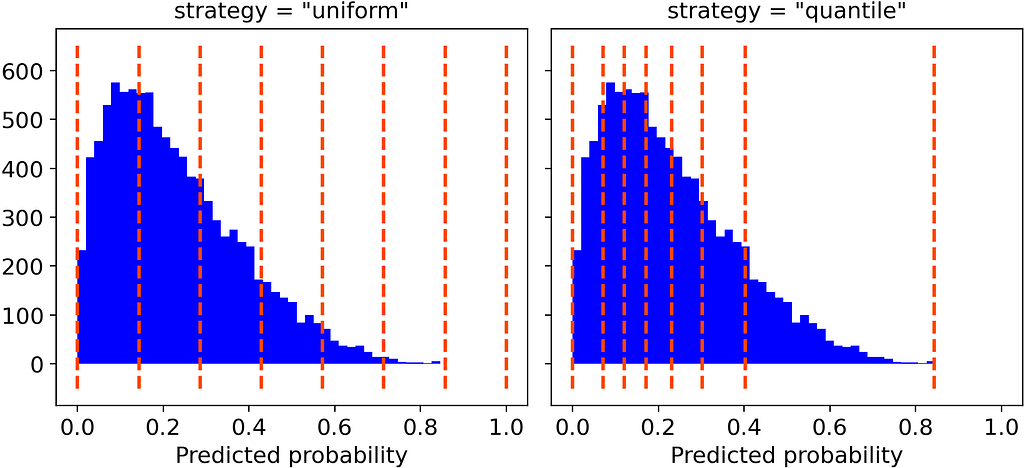Strategies of binning, with number of bins = 7. [Image by Author]

For plotting purposes, I personally prefer the “quantile” approach. In fact, “uniform” binning may be misleading because some bins could contain very few observations.

The Numpy function returns two arrays containing, for each bin, the mean probability and the mean of the target variable. Therefore, all we need to do is to plot them:

`from matplotlib.pyplot as plt`
`plt.plot([0, 1], [0, 1], linestyle = '--', label = 'Perfect calibration')plt.plot(proba_means, y_means)`

Supposing that your model has a good precision, the calibration curve will be monotonically increasing. But this doesn’t mean that the model is well calibrated. Indeed, your model is well calibrated only if the calibration curve is very close to the bisector (i.e. the dashed grey line), since this would mean that the predicted probability is on average close to the theoretical probability.

Let’s see some examples of common types of calibration curves that indicate a miscalibration of your model: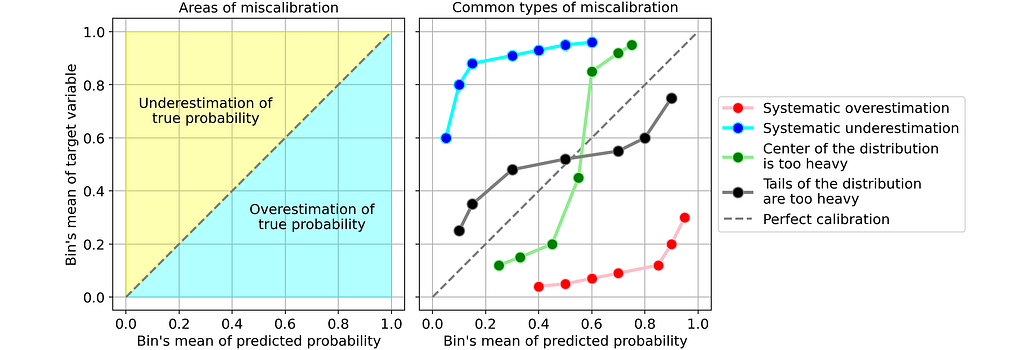Common examples of miscalibration. [Image by Author]

The most common types of miscalibration are:

• Systematic overestimation. Compared to the true distribution, the distribution of predicted probabilities is pushed towards the right. This is common when you train a model on an unbalanced dataset with very few positives.
• Systematic underestimation. Compared to the true distribution, the distribution of predicted probabilities is pushed leftward.
• Center of the distribution is too heavy. This happens when “algorithms such as support vector machines and boosted trees tend to push predicted probabilities away from 0 and 1” (quote from «Predicting good probabilities with supervised learning»).
• Tails of the distribution are too heavy. For instance, “Other methods such as naive bayes have the opposite bias and tend to push predictions closer to 0 and 1” (quote from «Predicting good probabilities with supervised learning»).

### How to fix miscalibration (in Python)

Suppose you have trained a classifier that yields accurate but uncalibrated probabilities. The idea of probability calibration is to build a second model (called calibrator) that is able to “correct” them into real probabilities.

Note that calibration should not be carried out on the same data that has been used for training the first classifier.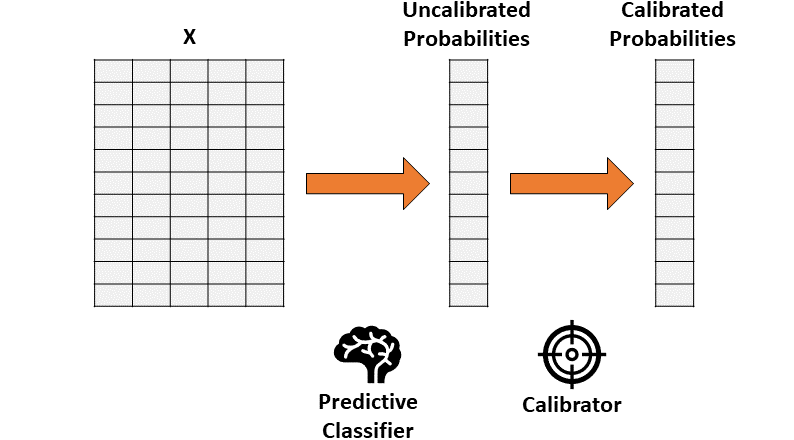Probability calibration through a two-step approach. [Image by Author]
Therefore, calibration consists in a function that transforms a 1-dimensional vector (of uncalibrated probabilities) into another 1-dimensional vector (of calibrated probabilities).

Two methods are mostly used as calibrators:

• Isotonic regression. A non-parametric algorithm that fits a non-decreasing free form line to the data. The fact that the line is non-decreasing is fundamental because it respects the original sorting.
• Logistic regression.

Let’s see how to use calibrators in practice in Python, with the help of a toy dataset:

`from sklearn.datasets import make_classification`
`X, y = make_classification(    n_samples = 15000,     n_features = 50,     n_informative = 30,     n_redundant = 20,    weights = [.9, .1],    random_state = 0)`
`X_train, X_valid, X_test = X[:5000], X[5000:10000], X[10000:]y_train, y_valid, y_test = y[:5000], y[5000:10000], y[10000:]`

First of all, we will need to fit a classifier. Let’s use random forest (but any model that has a «predict_proba» method would be ok).

`from sklearn.ensemble import RandomForestClassifier`
`forest = RandomForestClassifier().fit(X_train, y_train)`
`proba_valid = forest.predict_proba(X_valid)[:, 1]`

Then, we will use the output of the classifier (on validation data) to fit the calibrator and finally predicting probabilities on test data.

• Isotonic regression:
`from sklearn.isotonic import IsotonicRegression`
`iso_reg = IsotonicRegression(y_min = 0, y_max = 1, out_of_bounds = 'clip').fit(proba_valid, y_valid)`
`proba_test_forest_isoreg = iso_reg.predict(forest.predict_proba(X_test)[:, 1])`
• Logistic regression:
`from sklearn.linear_model import LogisticRegression`
`log_reg = LogisticRegression().fit(proba_valid.reshape(-1, 1), y_valid)`
`proba_test_forest_logreg = log_reg.predict_proba(forest.predict_proba(X_test)[:, 1].reshape(-1, 1))[:, 1]`

At this point we have three options for predicting probabilities:

1. plain random forest,
2. random forest + isotonic regression,
3. random forest + logistic regression.

But how do we assess which one is the most calibrated?

### Quantifying miscalibration

Everybody likes plots. But besides the calibration plot, we need a quantitative way to measure (mis)calibration. The most commonly used metric is called Expected Calibration Error. It answers the question:

How far away is our predicted probability from the true probability, on average?

Let’s take one classifier for instance: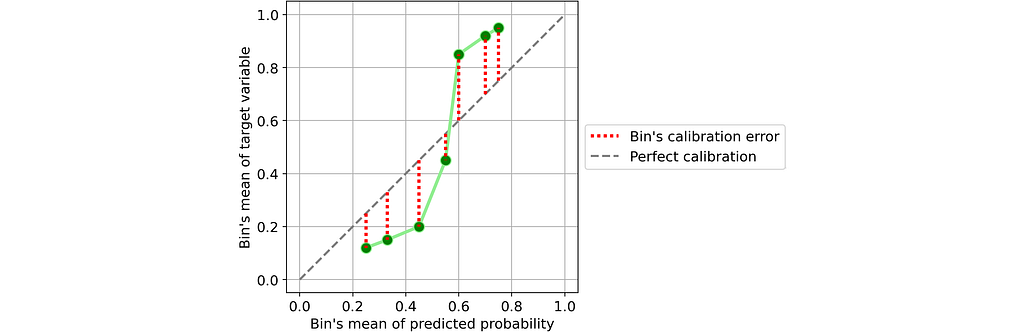Calibration of single bins. [Image by Author]

It’s easy to define the calibration error of a single bin: it’s the absolute difference between the mean of predicted probabilities and the fraction of positives within the same bin.

If you think about it, it’s pretty intuitive. Take one bin, and suppose the mean of its predicted probabilities is 25%. Thus, we expect that the fraction of positives in that bin is approximately equal to 25%. The farther this value is from 25%, the worse the calibration of that bin.

Thus, Expected Calibration Error (ECE) is a weighted mean of the calibration errors of the single bins, where each bin weighs proportionally to the number of observations that it contains:Expected Calibration Error. [Image by Author]

where b identifies a bin and B is the number of bins. Note that the denominator is simply the total number of samples.

But this formula leaves us the problem of defining the number of bins. In order to find a metric that is as neutral as possible, I propose to set the number of bins according to the Freedman-Diaconis rule (which is a statistical rule designed for finding the number of bins that makes the histogram as close as possible to the theoretical probability distribution).

Using Freedman-Diaconis rule in Python is extremely simple because it’s already implemented in numpy’s histogram function (it’s enough to pass the string “fd” to the parameter “bins”).

Here is a Python implementation of the expected calibration error, which employs the Freedman-Diaconis rule as default:

`def expected_calibration_error(y, proba, bins = 'fd'):`
`  import numpy as np`
`  bin_count, bin_edges = np.histogram(proba, bins = bins)  n_bins = len(bin_count)`
`  bin_edges -= 1e-8 # because left edge is not included  bin_id = np.digitize(proba, bin_edges, right = True) - 1`
`  bin_ysum = np.bincount(bin_id, weights = y, minlength = n_bins)  bin_probasum = np.bincount(bin_id, weights = proba, minlength = n_bins)`
`  bin_ymean = np.divide(bin_ysum, bin_count, out = np.zeros(n_bins), where = bin_count > 0)  bin_probamean = np.divide(bin_probasum, bin_count, out = np.zeros(n_bins), where = bin_count > 0)`
`  ece = np.abs((bin_probamean - bin_ymean) * bin_count).sum() / len(proba)`
`  return ece`

Now that we have a metric for calibration, let’s compare the calibration of the three models that we have obtained above (on test set):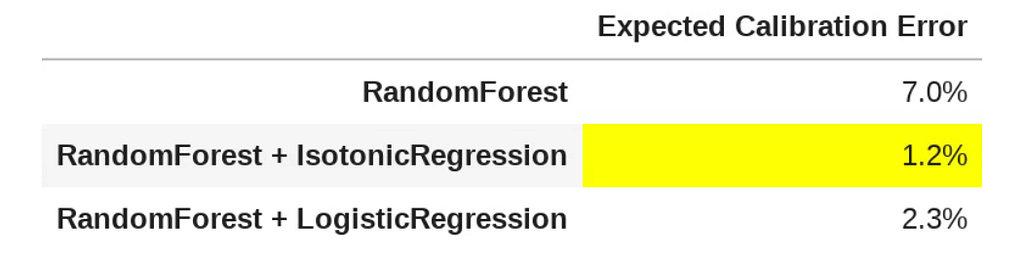Expected calibration error of models. [Image by Author]

In this case, isotonic regression provided the best outcome in terms of calibration, being far only 1.3% from the true probability, on average. It’s a huge improvement, if you consider that the ECE of the plain random forest was 7.4%.

### Reference

Here are some interesting papers (on which this article is based) that I recommend if you want to deepen the topic of probability calibration:

Thank you for reading! I hope you found this post useful.

I appreciate feedback and constructive criticism. If you want to talk about this article or other related topics, you can text me at my Linkedin contact.Python’s «predict_proba» Doesn’t Actually Predict Probabilities (and How to Fix It) was originally published in Towards Data Science on Medium, where people are continuing the conversation by highlighting and responding to this story.